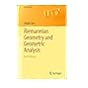Normal view

# Riemannian Geometry and Geometric Analysis [electronic resource] / by Jürgen Jost.

Material type:TextSeries: UniversitextPublisher: Berlin, Heidelberg : Springer Berlin Heidelberg, 2008Description: XIV, 590 p. 14 illus., 4 illus. in color. online resourceContent type: text Media type: computer Carrier type: online resourceISBN: 9783540773412Additional physical formats: Printed edition:: No titleDDC classification: 516.36 LOC classification: QA641-670Online resources: Click here to access online
Contents:
Foundational Material -- De Rham Cohomology and Harmonic Differential Forms -- Parallel Transport, Connections, and Covariant Derivatives -- Geodesics and Jacobi Fields -- Symmetric Spaces and Kähler Manifolds -- Morse Theory and Floer Homology -- Harmonic Maps between Riemannian Manifolds -- Harmonic maps from Riemann surfaces -- Variational Problems from Quantum Field Theory.
Summary: This established reference work continues to lead its readers to some of the hottest topics of contemporary mathematical research.This new edition introduces and explains the ideas of the parabolic methods that have recently found such a spectacular success in the work of Perelman at the examples of closed geodesics and harmonic forms. It also discusses further examples of geometric variational problems from quantum field theory, another source of profound new ideas and methods in geometry. From the reviews: "This book provides a very readable introduction to Riemannian geometry and geometric analysis. The author focuses on using analytic methods in the study of some fundamental theorems in Riemannian geometry, e.g., the Hodge theorem, the Rauch comparison theorem, the Lyusternik and Fet theorem and the existence of harmonic mappings. With the vast development of the mathematical subject of geometric analysis, the present textbook is most welcome. [..] The book is made more interesting by the perspectives in various sections." Mathematical Reviews  .
Tags from this library: No tags from this library for this title.
Star ratingsAverage rating: 0.0 (0 votes)
Holdings
Item type Current library Collection Call number Status Date due Barcode Item holdseBook e-Library

Electronic Book@IST

EBook Available
Total holds: 0

Foundational Material -- De Rham Cohomology and Harmonic Differential Forms -- Parallel Transport, Connections, and Covariant Derivatives -- Geodesics and Jacobi Fields -- Symmetric Spaces and Kähler Manifolds -- Morse Theory and Floer Homology -- Harmonic Maps between Riemannian Manifolds -- Harmonic maps from Riemann surfaces -- Variational Problems from Quantum Field Theory.

This established reference work continues to lead its readers to some of the hottest topics of contemporary mathematical research.This new edition introduces and explains the ideas of the parabolic methods that have recently found such a spectacular success in the work of Perelman at the examples of closed geodesics and harmonic forms. It also discusses further examples of geometric variational problems from quantum field theory, another source of profound new ideas and methods in geometry. From the reviews: "This book provides a very readable introduction to Riemannian geometry and geometric analysis. The author focuses on using analytic methods in the study of some fundamental theorems in Riemannian geometry, e.g., the Hodge theorem, the Rauch comparison theorem, the Lyusternik and Fet theorem and the existence of harmonic mappings. With the vast development of the mathematical subject of geometric analysis, the present textbook is most welcome. [..] The book is made more interesting by the perspectives in various sections." Mathematical Reviews  .

## Other editions of this workRiemannian Geometry and Geometric Analysis (Universitext). by Jost, Jürgen. ©2011
Share